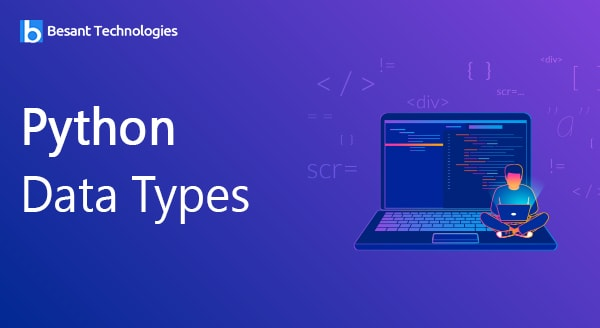Don't let the Lockdown slow you Down - Enroll Now and Get 3 Course at 24,999/- Only. Explore Now!

# General# Python Data Types

## Python Data Types

Python contains five standard data types as listed

• Numbers (int,long,float,complex)
• String
• List
• Tuple
• Dictionary

### Numbers (int,long,float,complex)

Made by the python variable method, python normally converts numbers from one type to another as per the requirement but python conversion functions like complex, long, float and int can also be used.

Example:

```integer value
a=200
float value
b=134.2388
Complex value
c=300+3k```

### String

When a string is chosen, Python recognizes it as per the double or single quotes entered around the text. Strings are normally used to display the written program.

### List

Lists are a Python version of arrays in other programming languages. Lists can be different always making it a key tool of Python. Implementation of stacks and queues is one of the most important functions of list and they are alterable.

Example:

only strings

```b=["Enjoy","your","Life"]
print(b)```

list of having both integers and strings

```p= ["We","have",2,"go keep on learning"]
print(p)```

### Tuple

Tuple and list are very much similar apart from the difference that a Tuple cannot be amended upon implementation.

Example:

Tuple having both integers and strings.

```p= ("We","have",2,"go keep on learning")
Print (p)```

### Dictionary

Python dictionary is a non-sequential set of items. Having a key as a value pair is what differentiates dictionary from other data types.

Example:

p = {1:”EmpName”,”EmpDept”:”Development”, “Salary”:33000} print value which key is 1 Print (p)